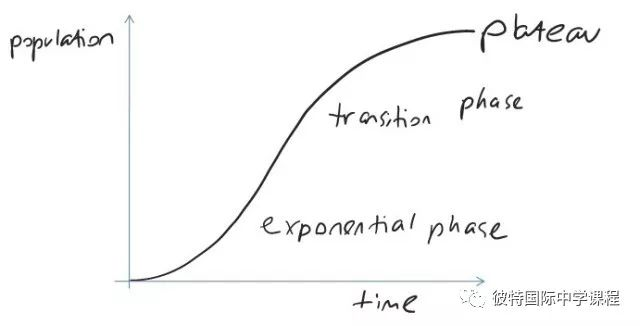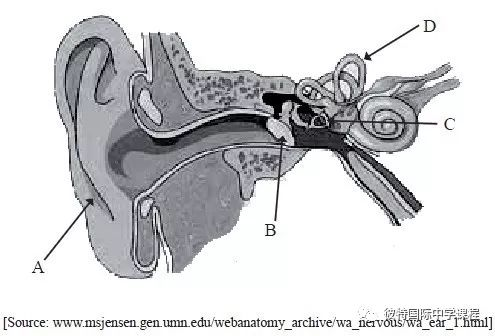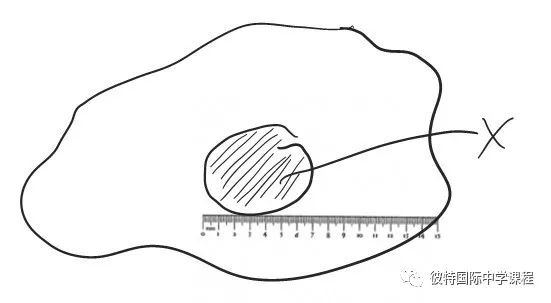# Command Terms in IB Biology--------上

12

Command Terms in IB BiologyAll definitions of command terms are taken from the IB Biology Subject Guide:

All IB Biology questions and assessment statements are built around  these

command terms, which let you know exactly what is expected of  you.

They are grouped according to the objectives of IB Biology:

Define         Measure            Draw

Label           List                     State

Objective 1: Demonstrate an understanding of:

-scientific facts and concepts

-scientific methods and        techniques

-scientific terminology

-methods of presenting scientific information

Distinguish        Describe

Identify                Calculate

Estimate            Apply

Annotate            Outline

Objective 2: Apply and Use:

-scientific facts and concepts

-scientific methods and techniques

-scientific terminology to communicate  effectively

-appropriate methods of presenting  scientific  information

Suggest         Construct

Comment       Explain

Solve               Determine

Design           Compare

Derive             Predict

Discuss          Sketch

Deduce           Show

Evaluate         Analyse

Objective 3: Construct, Analyse and Evaluate:

-hypotheses, research questions and  predictions

- scientific methods and techniques

-scientific explanations

Suggestions

•  Use the sample questions as a revision test:

- try to answer each question on scrap paper before showing the answer key

• Make up a collection of questions for each command term:

- use past papers, the syllabus and the QuestionBank CD Rom.

Define

"Give the precise meaning of a word, phrase or physical quantity."

Example

“Define diffusion and osmosis.”

Diffusion is the passive movement of particles from regions of high concentration to lower concentration.

Osmosis is the passive movement of water molecules, across a partially (selectively) permeable membrane, from a region of lower solute concentration to a region of higher solute concentration.

Tips:

• Definitions are in the subject guide

• Break the definition into its component parts – this will help with explanations

• Make up a vocab list or use an online glossary to help with define questions

Draw

"Represent by means of pencil lines."

Example:

“Draw a labeled graph showing a typical sigmoidal population growth curve ”Tips:

• Draw using clear, dark pencil lines (no colours)

• Pay attention to whether it needs to be labeled or annotated

• Make up drawings, graphs and diagrams revision book, and test each other

Label

"Add labels to a diagram."

Example:

“Label the structures of the human ear.”A = pinna

B = eardrum

C = stapes/ bones of middle ear

D =  semicircular canals

Tips:

• Generally, two correct labels are worth one mark

• Make up drawings, graphs and diagrams revision book, and test each other

• Revise and make links with regard to structures and their functionsList

"Give a sequence of names or other brief answers with no explanation."

Example:

“List seven levels in the hierarchy of taxa”

Kingdom, phylum, class, order, family, genus, species

“List two examples of fibrous proteins”

Keratin, collagen

Tips:

• Lists can be used to present examples of any of the assessment statements

• Use mnemonics for memory where the order of the list is important

• Try to use examples that can link topics together, across the course

Measure

"Find a value for a quantity."

Example:

“Measure the length of organelle x.”Tips:

• Bring a ruler to the exam !

• Generally, you’ll need to calculate from a measurement, rather than measure directly

• Present all answers in metric, SI units

State

"Give a specific name, value or other brief answer without an explanation or calculation."

Example:

“State which species is most distantly related to T. perkinsi on the basis of the tree diagram.”Tips:

• Definitions are in the subject guide

• Break the definition into its component parts – this will help with explanations

• Make up a vocab list or use an online glossary to help with define questions